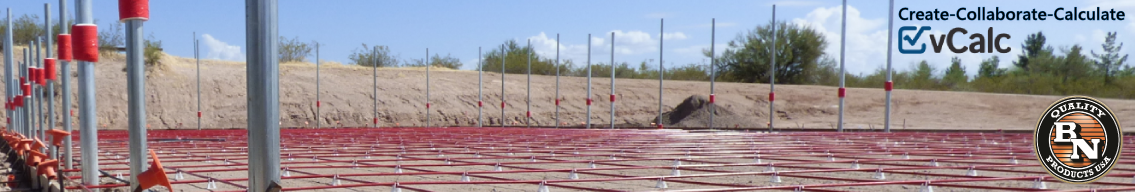# Rebar in a Circular SlabThe Rebar in a Circular Shaped Slab calculator estimates the total length and weight of reinforcement bars (rebar) needed for a circular shaped concrete slab.

INSTRUCTIONS: Choose units and enter the following:

• (r) Radius of Circular Slab (e.g. 12 ft).
• (D) Depth of Slab (e.g. 6″)
• (i) Inset from the edge of the circular slab (e.g. 3 inches)
• (oCon-center spacing of the rebar.
• (rS) Rebar Size
• (LF) Lapping Factor
• (M) Number of rebar mats
• (rL) un-cut Rebar Length
• (WL) Output choice:  Choose rebar weight or length

Weight and Length of Rebar in a Circular Slab: The calculator returns the:

• Length of rebar in feet
• Weight rebar in pounds
• Surface Area of Slab in square feet
• Total Volume of the Slab in cubic yards
• Volume of Concrete in cubic yards
• Concrete Displaced by Rebar in cubic yards

However, these can be automatically converted to compatible units (e.g. meters or kilograms) via the pull-down menu.